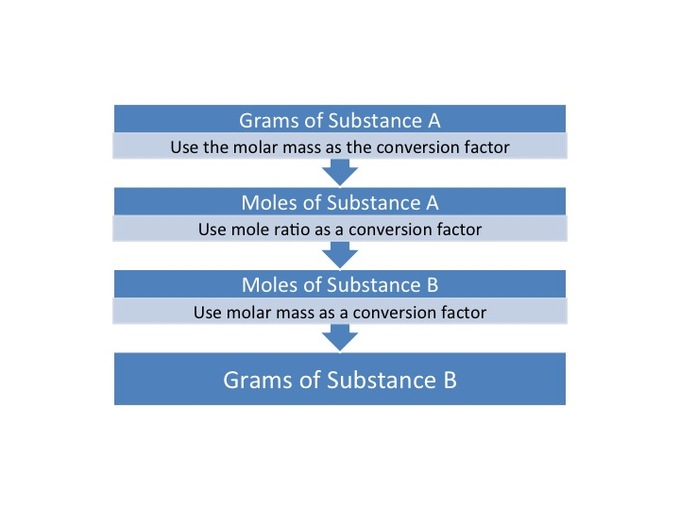## Mass-to-Mass Conversions

#### Learning Objective

• Calculate the mass of reactants and products from a balanced chemical equation and information about the amount of reactant(s) present.

#### Key Points

• The law of conservation of mass dictates that the quantity of an element does not change over the course of the reaction. Therefore, a chemical equation is balanced when each element has equal numbers on both the left and right sides of the equation.
• Stoichiometric ratios, the ratios of the amounts of each substance used, are unique for each chemical reaction.
• The balanced equation of a reaction contains the stoichiometric ratios of the reactants and products; these ratios can be used for mole-to-mole conversions. There is no direct way to convert from the mass of one substance to the mass of another.
• To convert from one mass (substance A) to another mass (substance B), you must convert the mass of A first to moles, then use the mole-to-mole conversion factor (B/A), then convert the mole amount of B back to grams of B.

#### Term

• stoichiometric ratioThe quantitative ratio between the reactants and products of a specific reaction or chemical equation. The ratio is made up of their coefficients from the balanced equation.

A chemical equation is a visual representation of a chemical reaction. A typical chemical equation follows the form

$aA +bB \rightarrow cC +dD$

where an arrow separates the reactants on the left and the products on the right. The coefficients before the reactants and products are their stoichiometric values.

## Calculating the Mass of Reactants & Products

One may need to compute the mass of a reactant or product under certain reaction conditions. To do this, it is necessary to ensure that the reaction is balanced. The ratio of the coefficients of two of the compounds in a reaction (reactant or product) can be viewed as a conversion factor and can be used to facilitate mole-to-mole conversions within the reaction. It is not possible to directly convert from the mass of one element to the mass of another. Therefore, for a mass-to-mass conversion, it is necessary to first convert one amount to moles, then use the conversion factor to find moles of the other substance, and then convert the molar value of interest back to mass.Mass to mass conversionsA chart detailing the steps that need to be taken to convert from the mass of substance A to the mass of substance B.

## Example

This can be illustrated by the following example, which calculates the mass of oxygen needed to burn 54.0 grams of butane (C4H10). The balanced equation is:

$2C_{4}H_{10} + 13O_{2} \rightarrow 8CO_{2}+10H_{2}O$

Because there is no direct way to compare the mass of butane to the mass of oxygen, the mass of butane must be converted to moles of butane:

$54.0\ g \ C_4H_{10} \cdot \frac{1mol}{58.1 g} = 0.929\ mol \ C_4H_{10}$

With the number of moles of butane equal to 54 grams, it is possible to find the moles of O2 that can react with it. Taking coefficients from the reaction equation (13 O2 and 2 C4H10), the molar ratio of O2 to C4H10 is 13:2.

$0.929 \ mol \ C_4H_{10} \cdot \frac {13 \ mol \ O_2}{2\ mol \ C_4H_{10}} = 6.05\ mol \ O_2$

This last equation shows that 6.05 moles of O2 can react with 0.929 moles of C4H10. The molar amount of O2 can now be easily converted back to grams of oxygen:

$6.05 \ mol \cdot \frac{32\ g}{1 mol} = 193 \ g \ O_2$

In summary, it was impossible to directly determine the mass of oxygen that could react with 54.0 grams of butane. But by converting the butane mass to moles (0.929 moles) and using the molar ratio (13 moles oxygen : 2 moles butane), one can find the molar amount of oxygen (6.05 moles) that reacts with 54.0 grams of butane. Using the molar amount of oxygen, it is then possible to find the mass of the oxygen (193 g).